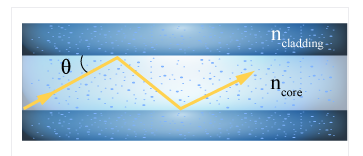# Problem:  Fiber optics are an important part of our modern internet. In these fibers, two different glasses are used to confine the light by total internal reflection at the critical angle for the interface between the core (ncore = 1.482 ) and the cladding (ncladding = 1.44).Suppose you wanted the largest angle at which total internal reflection occurred to be θmax = 5 degrees. What index of refraction does the cladding need if the core is unchanged

###### FREE Expert Solution

If θmax = 5°

α1 = 90° - 5° = 85°

86% (372 ratings)###### Problem Details

Fiber optics are an important part of our modern internet. In these fibers, two different glasses are used to confine the light by total internal reflection at the critical angle for the interface between the core (ncore = 1.482 ) and the cladding (ncladding = 1.44).

Suppose you wanted the largest angle at which total internal reflection occurred to be θmax = 5 degrees. What index of refraction does the cladding need if the core is unchangedFrequently Asked Questions

What scientific concept do you need to know in order to solve this problem?

Our tutors have indicated that to solve this problem you will need to apply the Refraction Of Light concept. You can view video lessons to learn Refraction Of Light. Or if you need more Refraction Of Light practice, you can also practice Refraction Of Light practice problems.